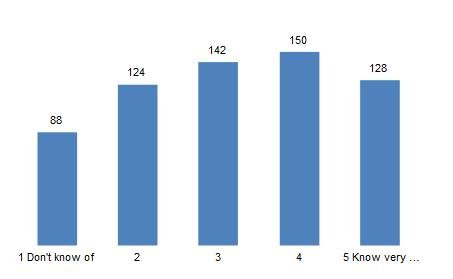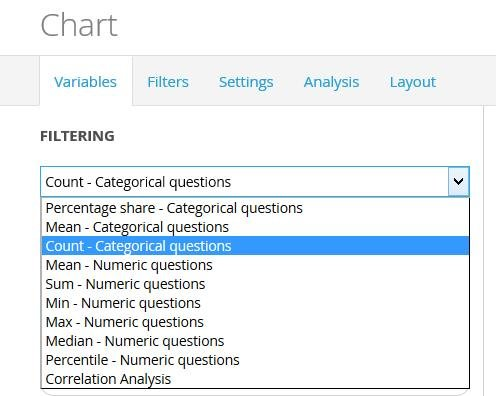The ability to calculate and show the count per answer alternative of categorical questions is present for charts and tables in the StoryTeller. The count can also be used in the Dynamic icons/shapes objects. Like all other calculation types, the count result can be based on weighted or unweighted data. The image below shows an example of a chart where Count is used.The Count calculation can be found in the ordinary calculation type list, as shown in the image below. The rest of the setup of a Chart, Table and Dynamic Icons/Shapes is more or less identical to setting up an object with a percent calculation.You are not able to use the significance testing calculations when Count is selected. The significance test calculation can only be applied to Mean and Percentage calculations of categorical questions.